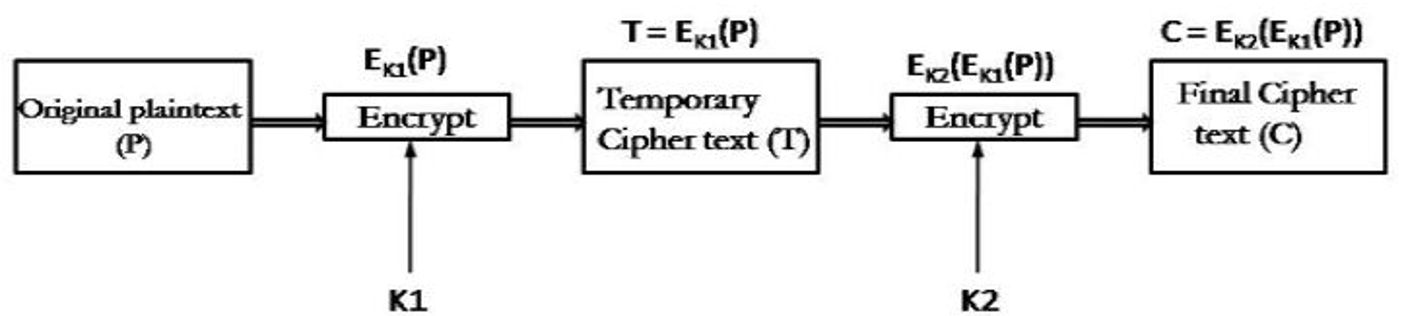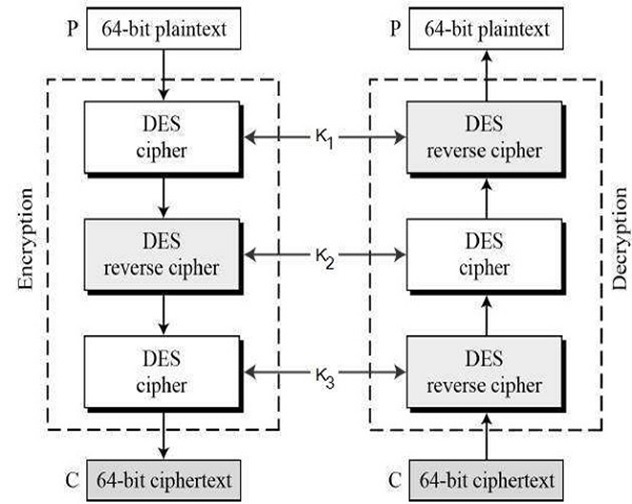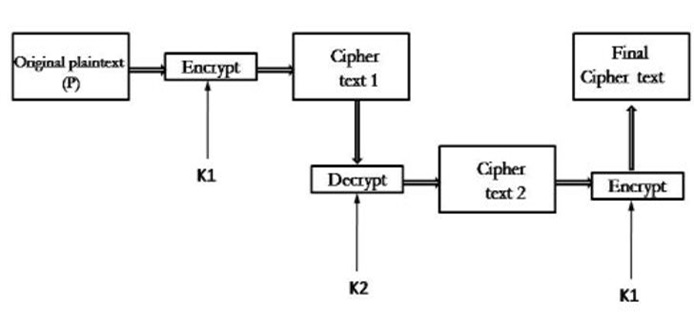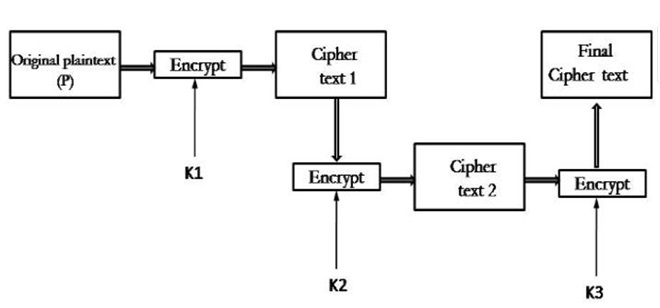# Multiple DES in Cryptography

Cryptography | Multiple DES: In this tutorial, we will learn about Multiple DES, and their steps of encryption and decryption. By Monika Sharma Last updated : May 25, 2023

This is a DES that was susceptible to attacks due to tremendous advances in computer hardware in cryptography. Hence, it was a very complex or competent algorithm it would be feasible to reuse DES rather than writing an of cryptography.

Required to this variation of DES were introduced known as multiple DES which was as follows as Shown:

## 1) Double DES

• Mainly, Double DES is simple as it does that normal DES does. Double DES uses twp keys to say K1 and K2 in this algorithm. It first performs DES on the original plain text using K! to get the encrypted text in cryptography. Here, it again performs DES on the encrypted text but this time with the other key K2 in this algorithm.
• Firstly, the final output is the encryption of encrypted text with the original plain text encrypted twice with two different keys shown in the structure as given below:• While the doubly encrypted ciphertext block is first decrypted using the key K2 to produce singly encrypted ciphertext by plaintext or original text. Hence, this ciphertext block is then decrypted using the key K1 to obtain the original plain text block in cryptography.
• Mainly, the cryptanalysis for the basic version of DES requires a search of 256 thus the assumption is Double DES require 2128 keys which is not true for the message.
Here, a Meet-in-the-middle attack is the drawback of double DES in this. Mainly, this attack involves encryption from one end, decryption from the other and matching the results in the middle hence the name in the message.

Therefore, the simplest form of multiple encryptions has two encryption stages and two keys in this. Here, given a plaintext P and two encryption keys K1 and K2, ciphertext C is created as: C = E(K2, E(K1, P))

Then, Decryption requires that the keys be applied in reverse order as: P = D(K1, D(K2, C))

Now, For DES, this scheme involves a key length of 56 * 2 = 112 bits, resulting in a dramatic increase in cryptographic strength in this. Further, but we need to examine the algorithm more closely for this.

## 2) Triple DES

Here, to improve the security of DES to a higher level triple DES was proposed in this. While this uses three stages on DES for encryption and decryption in cryptography.There basically of two versions of triple-DES are as given:

### 2.1) Triple DES with Two Keys

• While in triple DES with two keys there are only two keys K1 used by the first and third stages and K2 used in the second stage in this. Basically, first, the plain text is encrypted with key K1 then the output of step one is decrypted with K2 and final the output second step is encrypted again with key K1 in cryptography.
•• It is also called encrypt decrypt encrypt (ECE) mode in cryptography. Hence, Triple DES with two keys is not susceptible to the meet-in-the-middle attack in cryptography.

### 2.2) Triple DES with Three Keys

• It basically had the idea of Triple-DES with three keys:
•• While the plain text block P is first encrypted with a key K1 then encrypts with a second key K2 and finally with a third key K3 where K1, K2, and K3 are all different from each other in this algorithm. This is Decryption is done in reverse order in this way. Therefore, this algorithm is mostly used in PGP and S/MIME in cryptography.
• Therefore, the DES cipher's key size of multiple of 56 bits of the message was generally enough when that algorithm was designed but the availability of increasing computational power made brute force attacks feasible in this algorithm. Thus, the Triple-DES provides a relatively simple method of increasing the key size of DES to secure against such attacks like that, without the need to design a completely new block cipher algorithm by this technique in cryptography.

## MEET-IN-THE-MIDDLE ATTACK

Therefore, the use of double DES results in a mapping that is not equivalent to a single DES encryption in cryptography. Hence, but there is a way to attack this scheme, one that does not depend on any particular property of DES but that will work against any block encryption cipher in cryptography.

Therefore, the algorithm known as a meet-in-the-middle attack was first described in [DIFF77]. It is based on the observation that we can say if we have it

Here, it has given a known pair, (P, C), the attack proceeds as follows as see. Firstly, encrypt P for all 256 possible values of K1 in this. While storing these results in a table and then sort the table by the values of X. Next, decrypt C using all 256 possible values of K2 in cryptography. Therefore, as each decryption is produced, check the result against the table for a match by this. Either if a match occurs, then test the two resulting keys against a new known-plaintext–ciphertext pair in cryptography. Hence, if the two keys produce the correct ciphertext, accept them as the correct keys in this algorithm.

For Example, for any given plaintext or original text P, 264 possible ciphertext values could be produced by double DES in this. This was Double DES uses, in effect, a 112-bit key, so that there are 2112 possible keys apply to messages. Therefore, on mean, for a given plaintext or original text P, the number of different 112-bit keys that will produce a given ciphertext as C is given: 2112/264 = 248

While, the foregoing procedure will produce about 248 false alarms on the first (P, C) pair in this. Therefore, a similar argument indicates that with an additional 64 bits of known plaintext and ciphertext, the false alarm rate is reduced to 248 - 64 = 2 - 16 in mathematics. While putting another way, if the meet-in-the-middle attack is performed on two blocks of known plaintext–ciphertext, the probability that the correct keys are determined is 1 - 2 - 16 in this way. Thus, the result is that a known-plaintext attack will succeed against double DES, which has a key size of 112 bits, with an effort on the order of 256, which is not much more than the 255 required for single DES in cryptography.

Image source: https://www.tutorialspoint.com/cryptography/triple_des.htm